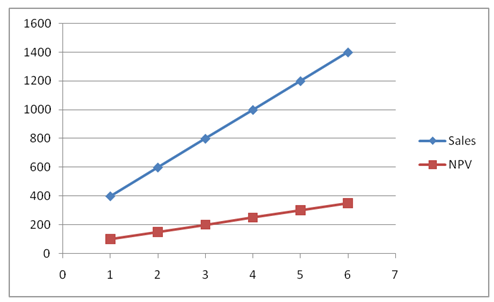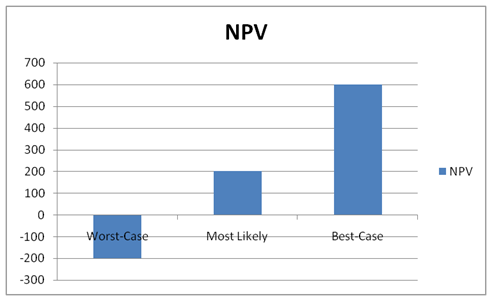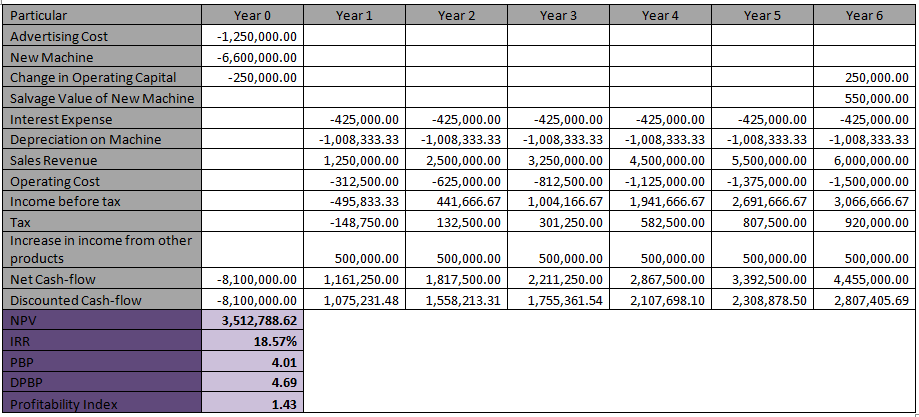# FIN200 Corporate Financial Management Proof Reading Services## Question 1

### Introduction

Capital Budgeting is one of the widely used techniques for new investments making decision. It considers the complete picture of investment opportunities in terms of cash-flows of the investment over the life of the project or investment. However, it must be noted that the capital budgeting techniques are totally based on forecast and estimates. The economic and market conditions are always uncertain and you can not predict anything with 100% accuracy. In such an uncertain situation, sensitivity analysis and scenario analysis are used to measure the risk associated with the project if some of the variables are lower or higher than the forecasted value. In this report, we will try to understand the terms i.e. Sensitivity Analysis and Scenario Analysis and link them to the Capital Budgeting.#### Sensitivity Analysis

Sensitivity analysis is a tool to examine how sensitive the cash-flows of a project are to one of the variables. The question of doing sensitivity analysis arises after the cash-flow analysis is completed with base forecasts. Once the base forecast is ready, one of the variables i.e. component of cash-flows of the project is taken up for the analysis. The sensitivity analysis with that variable is done by changing the forecast value of the variable and measuring the impact of the change in the value of the variable to the final outcome of the project. (Cacuci, 2005)In the above graph, the sensitivity of the project with respect to sales value is replicated. The value of the sales (variable) is changed and accordingly the NPV of the project is calculated with the new values. The graph shows the value of the NPV of the project for different values of sales. As it can be seen in the above graph, the NPV of the project is quite sensitive to the sales forecast of the project. With increase in sales, the NPV of the project is increasing constantly. Thus, before undertaking the project, the company must ensure that it has got its forecast for sales as accurate as possible.

The sensitivity analysis can be done on any of the variable of the project like required rate of return (i.e. discounting factor), initial investment, tax rate, etc.

#### Scenario Analysis

The objective of Scenario analysis is quite similar to that of Sensitivity analysis. While sensitivity analysis measures the risk associated with the project, the Scenario analysis also does the same. Sensitivity analysis is done with only one variable, while scenario analysis is actually a combination of various variables. So, Scenario analysis is multiple sensitivity analysis. (Aaker, 2001)

In Scenario analysis, various scenarios of future are considered e.g. best-case scenario, most likely scenario and worst-case scenario. For all these scenarios, the forecast is adjusted accordingly and NPV is calculated differently for each scenario. Further step can be added by identifying probability of each scenario and then doing weighted average of all the NPVs and their probabilities.As it can be seen from the above graph, for different scenarios, different NPV values are replicated. The NPV of the project is negative in one of the scenarios i.e. worst-case, while it is positive in other two scenarios. Further analysis can also be done by assigning probability to each of these scenarios and calculating weighted average of the NPV.

#### Capital Budgeting

The capital budgeting involves calculating cash-flows of the project based on the base forecast of various components of cash-flows. There are various tools used in capital budgeting like NPV, Internal Rate of Return, Payback Period, Discounted Payback Period, Profitability Index, etc. However, all these tools measures the viability of the project based on the base forecast values. It does not consider the probability of changes in the forecast values.

So, the above two terms i.e. sensitivity analysis and scenario analysis, shows that the capital budgeting does not end by simply calculating NPV of the project or any other tool like IRR, Payback Period, etc. Capital budgeting is not that simple. Investors can not simply calculate NPV and if it is positive, go ahead with the project. They have to test the accuracy of their forecast and the impact of changes in the forecast values to the final outcome of the project. This is the point where sensitivity analysis and scenario analysis comes into the picture and this is the linking point where the capital budgeting and these analysis comes together.

The sensitivity analysis indicates the variables which need to be forecasted accurately and which are not of much importance to the outcome of the project. Scenario analysis presents the picture of the project in different future uncertain events. Thus, capital budgeting combined with sensitivity and scenario analysis gives a much better picture of the project to the investors and they can take better informed decision.

### Question 2

Before doing the calcuations for cash-flows and other measures of capital budgeting, it is important to note down the assumptions made to calculate the cash-flows. Below are the assumptions made to calculate the NPV of the project:

• Six month ago company paid some amount for research regarding the new product. This research expenses are not considered for the calculation of capital budgeting as this is sunk cost i.e. company already incurred it and it does not change if the company accepts or rejects the project.
• The effect in the net operating capital is given as \$250,000. However, it is not specified whether this is incremental increase or decrease. So, it is assumed that this amount is increase in the net operating capital if the company go ahead with the project.
• It is given that the company expects the increase in the sales of other products after tax with the introduction of this new product. It is not clear from the question that it is increase in sales after tax or income after tax. So, it is assumed that the amount given is the increase in income after tax from other products of the company due to introduction of this new product.
• The calculation of the cash-flows (given in the table below) shows that the tax amount for the first year of the project ius negative. As this is the tax on the income of just one product, it is assumed that the tax on the total income of the company (all the products) will be positive and the company will be able to save on the tax on the income of the other products due to introduction of this product. Otherwise, the calculation should consider the tax amount for the first year as zero (\$0).

Following table shows the detailed calculation of the cash-flows, NPV, IRR, Payback Period, Discounted Payback Period and Profitability Index:From the above analysis, following recommendation can be made regarding the viability of the project:

• The NPV of the project (\$3,512,788.12) is positive. As it is positive, the project should be accepted.
• The IRR of the project (18.57%) is very much above the required rate of the return i.e. 8%. So, the project should be accepted as per IRR measure as well.
• Payback period and discounted payback period are 4.01 and 4.69 respectively. There is no standard benchmark here, but this surely does not indicate bad investment.
• Profitability index of the project is 1.43. As it is more than one, the project should be accepted.

Overall, all the above measures of capital budgeting suggests a positive sign for the project. Therefore, Alborz Company should accept this project and go ahead with the introduction of the new product in the market.

### Conclusion

In conclusion, it can be said that capital budgeting is vague without sensitivity analysis and scenario analysis. This is because wrong forecast can easily mislead the decision maker by showing robust or worst picture of the cash-flows of the project. So, there arises the requirement to test the accuracy of the forecast and the impact of change in the variables to the cash-flows of the project. The sensitivity and scenario analysis are, thus, very useful tools to combine with capital budgeting. These tools ensure better and detailed information to the investors before making investment decisions.

### References

• Cacuci, Dan G. 2005, Sensitivity and Uncertainty Analysis: Theory, Chapman & Hall.
• Aaker, David A. 2001, Strategic Market Management. New York: John Wiley & Sons.